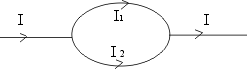In a region where there is an electric field, the electric forces do +8.0 x 10-19 J of work on an electron as it moves from point X to point Y. Which point, X or Y is at a higher potential? What is the potential difference, Vy - Vx , between point Y and point X?

W = qV

If W is positive, either both q and V are positive or both q and V are negative. Since electron ha a negative charge, V should be negative in this case (W is positive). Thus final potential must be less than the initial potential. Thus X is at a higher potential.

V = Vy - Vx

W = 8.0 x 10-19 J

q = -1.6 x 10-19 C

V = Vy - Vx = W/q = 8.0 x 10-19 / -1.6 x 10-19 = -5 Volt.

A parallel plate capacitor has a capacitance of 1.2nF. There is a charge of magnitude 0.800 ï¿½C on each plate. What is the potential difference between the plates? If the plate separation is doubled, while the charge is kept constant, what will happen to the potential difference?

C = 1.20 x 10-9 F

Q = 0.800 x 10-6 C

Q = CV

or V = Q/C = 0.800 x 10-6 / 1.20 x 10-9 = 0.667 x 10+3 V

The capacitance of a parallel plate capacitor

C = Aeo /d

where d is the plate seperation

If separation is doubled, C is halved.

If Q is kept constant, V = Q/C will be doubled.

In the circuit given below find the value of resistance R and then find the value of the maximum power to resistance R.Q The ac generator in Fig below supplies 120 V (rms) at 60 Hz. With the switch open as in the diagram, the resulting current leads the generator emf by 20 degs. With the switch in position 1 the current lags the generator emf by 10 deg. When the switch is in position 2 the rms current is 2.0 A. find the values of R,L, and c.Ev = 120V , f=60 Hz , w=2pif = 360 radians

Current I leads by 20 degs

Phase is negative, Xl < Xc

Tan (-20deg)= (Xl - Xc)/R = -0.36 ---- (1)

Or (Xc -Xl)/R =0.36

I lags by 10 degs and phase is positive

Xl > Xc1

Tan 10 degs = 0.18 = (Xl - Xc1)/R ------(2)

Z=Xc - Xl = Ev /Iv = 120/2 =60 ---------(3)

Substituting 3 in 1

60/R=0.36

R=60/0.36 = 500/3 ohms

Equation 2 implies wL-1/2wC = 0.06 x 500/3 = 30

Equation 3 implies 1/wC -wL=60 ----------(a)

wL = 30 + 1/2wC ------(b)

substituting in a

1/wC -30 - 1/2wC =60

1/2wC = 90 -------------(c)

C=0.15 ï¿½F

Substituting c in b

wL=30 + 90

L= 1/3 H

Q Find the magnetic field at the center of the loop shown.B = (ï¿½o/4pi)(IL/r2)

B = 0

Since I1R1 = I2R2 or I1L1 = I2L2

Or B = (ï¿½o/4pir2)( I1L1 - I2L2) = 0

Q What is the back emf in a motor that draws 7.1 A from a 220 volt line if the field coils have a resistance of 200 ohms and are wound in parallel with an armature with a resistance of 3 ohms?

I=7.1 A , v = 220 V , R1 = 200 ohms, R2 = 3 ohms

Let E = back emf

I =(V-E)/R

1/R =1/3 +1/200 ie R = 600/203

E = V - IR = 220 - 21 = 199 V

Q. A horizontal rod 2 meters long is released in a region where there is a uniform horizontal magnetic induction of 0.6 Tesla perpendicular to the rod. How far has the rod fallen when the induced emf is 120V.

E=Blu

120 = 0.6 x 2x u

u = 100 m/s

initially u = o finally u =100 m/s

v2 - u2 =2as = 2gh

h= (1002 -0)/(2x10) =500 m